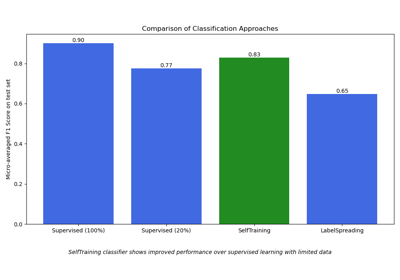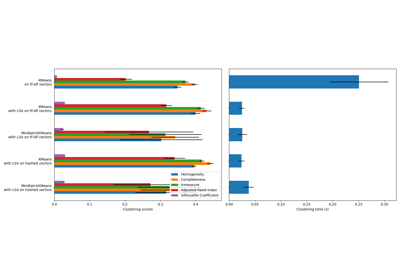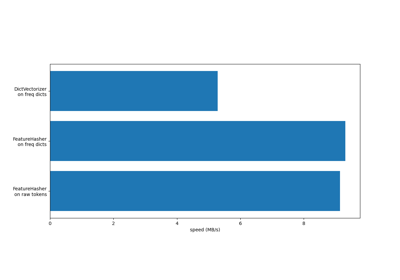# sklearn.feature_extraction.text.TfidfTransformer¶

class sklearn.feature_extraction.text.TfidfTransformer(*, norm='l2', use_idf=True, smooth_idf=True, sublinear_tf=False)[source]

Transform a count matrix to a normalized tf or tf-idf representation.

Tf means term-frequency while tf-idf means term-frequency times inverse document-frequency. This is a common term weighting scheme in information retrieval, that has also found good use in document classification.

The goal of using tf-idf instead of the raw frequencies of occurrence of a token in a given document is to scale down the impact of tokens that occur very frequently in a given corpus and that are hence empirically less informative than features that occur in a small fraction of the training corpus.

The formula that is used to compute the tf-idf for a term t of a document d in a document set is tf-idf(t, d) = tf(t, d) * idf(t), and the idf is computed as idf(t) = log [ n / df(t) ] + 1 (if smooth_idf=False), where n is the total number of documents in the document set and df(t) is the document frequency of t; the document frequency is the number of documents in the document set that contain the term t. The effect of adding “1” to the idf in the equation above is that terms with zero idf, i.e., terms that occur in all documents in a training set, will not be entirely ignored. (Note that the idf formula above differs from the standard textbook notation that defines the idf as idf(t) = log [ n / (df(t) + 1) ]).

If smooth_idf=True (the default), the constant “1” is added to the numerator and denominator of the idf as if an extra document was seen containing every term in the collection exactly once, which prevents zero divisions: idf(t) = log [ (1 + n) / (1 + df(t)) ] + 1.

Furthermore, the formulas used to compute tf and idf depend on parameter settings that correspond to the SMART notation used in IR as follows:

Tf is “n” (natural) by default, “l” (logarithmic) when sublinear_tf=True. Idf is “t” when use_idf is given, “n” (none) otherwise. Normalization is “c” (cosine) when norm='l2', “n” (none) when norm=None.

Read more in the User Guide.

Parameters:
norm{‘l1’, ‘l2’} or None, default=’l2’

Each output row will have unit norm, either:

• ‘l2’: Sum of squares of vector elements is 1. The cosine similarity between two vectors is their dot product when l2 norm has been applied.

• ‘l1’: Sum of absolute values of vector elements is 1. See preprocessing.normalize.

• None: No normalization.

use_idfbool, default=True

Enable inverse-document-frequency reweighting. If False, idf(t) = 1.

smooth_idfbool, default=True

Smooth idf weights by adding one to document frequencies, as if an extra document was seen containing every term in the collection exactly once. Prevents zero divisions.

sublinear_tfbool, default=False

Apply sublinear tf scaling, i.e. replace tf with 1 + log(tf).

Attributes:
idf_array of shape (n_features)

Inverse document frequency vector, only defined if use_idf=True.

n_features_in_int

Number of features seen during fit.

New in version 1.0.

feature_names_in_ndarray of shape (n_features_in_,)

Names of features seen during fit. Defined only when X has feature names that are all strings.

New in version 1.0.

CountVectorizer

Transforms text into a sparse matrix of n-gram counts.

TfidfVectorizer

Convert a collection of raw documents to a matrix of TF-IDF features.

HashingVectorizer

Convert a collection of text documents to a matrix of token occurrences.

References

[Yates2011]

R. Baeza-Yates and B. Ribeiro-Neto (2011). Modern Information Retrieval. Addison Wesley, pp. 68-74.

[MRS2008]

C.D. Manning, P. Raghavan and H. Schütze (2008). Introduction to Information Retrieval. Cambridge University Press, pp. 118-120.

Examples

>>> from sklearn.feature_extraction.text import TfidfTransformer
>>> from sklearn.feature_extraction.text import CountVectorizer
>>> from sklearn.pipeline import Pipeline
>>> corpus = ['this is the first document',
...           'this document is the second document',
...           'and this is the third one',
...           'is this the first document']
>>> vocabulary = ['this', 'document', 'first', 'is', 'second', 'the',
...               'and', 'one']
>>> pipe = Pipeline([('count', CountVectorizer(vocabulary=vocabulary)),
...                  ('tfid', TfidfTransformer())]).fit(corpus)
>>> pipe['count'].transform(corpus).toarray()
array([[1, 1, 1, 1, 0, 1, 0, 0],
[1, 2, 0, 1, 1, 1, 0, 0],
[1, 0, 0, 1, 0, 1, 1, 1],
[1, 1, 1, 1, 0, 1, 0, 0]])
>>> pipe['tfid'].idf_
array([1.        , 1.22314355, 1.51082562, 1.        , 1.91629073,
1.        , 1.91629073, 1.91629073])
>>> pipe.transform(corpus).shape
(4, 8)


Methods

 fit(X[, y]) Learn the idf vector (global term weights). fit_transform(X[, y]) Fit to data, then transform it. get_feature_names_out([input_features]) Get output feature names for transformation. get_params([deep]) Get parameters for this estimator. set_output(*[, transform]) Set output container. set_params(**params) Set the parameters of this estimator. transform(X[, copy]) Transform a count matrix to a tf or tf-idf representation.
fit(X, y=None)[source]

Learn the idf vector (global term weights).

Parameters:
Xsparse matrix of shape n_samples, n_features)

A matrix of term/token counts.

yNone

This parameter is not needed to compute tf-idf.

Returns:
selfobject

Fitted transformer.

fit_transform(X, y=None, **fit_params)[source]

Fit to data, then transform it.

Fits transformer to X and y with optional parameters fit_params and returns a transformed version of X.

Parameters:
Xarray-like of shape (n_samples, n_features)

Input samples.

yarray-like of shape (n_samples,) or (n_samples, n_outputs), default=None

Target values (None for unsupervised transformations).

**fit_paramsdict

Returns:
X_newndarray array of shape (n_samples, n_features_new)

Transformed array.

get_feature_names_out(input_features=None)[source]

Get output feature names for transformation.

Parameters:
input_featuresarray-like of str or None, default=None

Input features.

• If input_features is None, then feature_names_in_ is used as feature names in. If feature_names_in_ is not defined, then the following input feature names are generated: ["x0", "x1", ..., "x(n_features_in_ - 1)"].

• If input_features is an array-like, then input_features must match feature_names_in_ if feature_names_in_ is defined.

Returns:
feature_names_outndarray of str objects

Same as input features.

get_params(deep=True)[source]

Get parameters for this estimator.

Parameters:
deepbool, default=True

If True, will return the parameters for this estimator and contained subobjects that are estimators.

Returns:
paramsdict

Parameter names mapped to their values.

property idf_

Inverse document frequency vector, only defined if use_idf=True.

Returns:
ndarray of shape (n_features,)
set_output(*, transform=None)[source]

Set output container.

See Introducing the set_output API for an example on how to use the API.

Parameters:
transform{“default”, “pandas”}, default=None

Configure output of transform and fit_transform.

• "default": Default output format of a transformer

• "pandas": DataFrame output

• None: Transform configuration is unchanged

Returns:
selfestimator instance

Estimator instance.

set_params(**params)[source]

Set the parameters of this estimator.

The method works on simple estimators as well as on nested objects (such as Pipeline). The latter have parameters of the form <component>__<parameter> so that it’s possible to update each component of a nested object.

Parameters:
**paramsdict

Estimator parameters.

Returns:
selfestimator instance

Estimator instance.

transform(X, copy=True)[source]

Transform a count matrix to a tf or tf-idf representation.

Parameters:
Xsparse matrix of (n_samples, n_features)

A matrix of term/token counts.

copybool, default=True

Whether to copy X and operate on the copy or perform in-place operations.

Returns:
vectorssparse matrix of shape (n_samples, n_features)

Tf-idf-weighted document-term matrix.

## Examples using sklearn.feature_extraction.text.TfidfTransformer¶Semi-supervised Classification on a Text Dataset

Semi-supervised Classification on a Text DatasetClustering text documents using k-means

Clustering text documents using k-meansFeatureHasher and DictVectorizer Comparison

FeatureHasher and DictVectorizer Comparison